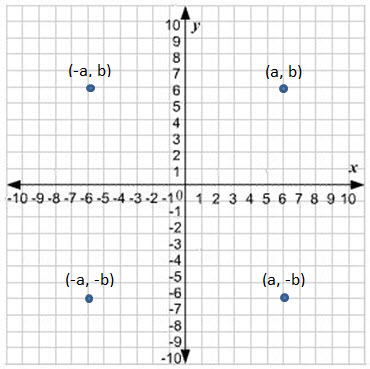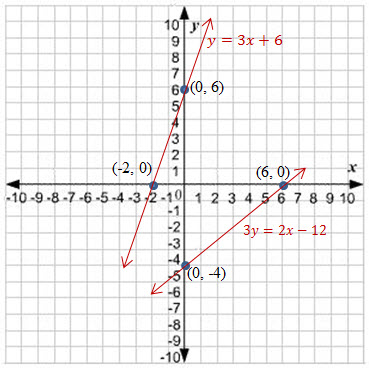Ordered Pair

An ordered pair$(a, b)$ is a pair of mathematical objects. The order in which the objects appear in the pair is significant: the ordered pair$(a, b)$ is different from the ordered pair$(b, a)$ unless$a=b$.

In the ordered pair$(a, b)$ , the object$a$ is called the$first \ entry$, and the object$b$ the$second \ entry$ of the pair.

Note$(a, b)$ is not equal to$(b, a)$. Hence$(4, 1)$ is not equal to$(1, 4)$.If$(a1, b1)$ and$(a2, b2)$ be ordered pairs, then$(a1, b1) \ and \ (a2, b2) \ only \ if \ a1=a2 \ and \ b1=b2$.

Coordinate System (two dimensional) or Cartesian Coordinate System

A Cartesian coordinate system is a coordinate system that specifies each point uniquely in a plane by a pair of numerical coordinates, which are the signed distances to the point from two fixed perpendicular directed lines, measured in the same unit of length.

Each reference line is called a coordinate axis or just axis of the system, and the point where they meet is its origin, usually at ordered pair$(0, 0)$The horizontal line is called the$x-axis$. The vertical line is called$y-axis$. The axis have a positive scale and a negative scale.

Each point on the plane is represented by an ordered pair say$(a, b)$$a$ is the distance on the$x-axis$ and$b$ is the distance on the$y-axis$ for the point .

Please refer to the above diagram for more clarity.  If you see, each point is represented by a pair of numbers, the first one is the distance of the point on the$x-axis$ and the second one is the distance of the point on the$y-axis$.The x coordinate is positive if it is measured to the right of
the origin and is negative if it is measured to the left of the origin.

Similarly, the y coordinate is positive if it is measured to above the origin and is negative if it is measure to below the origin.

The coordinate system is divided into four quadrants as shown in the figure.

A very important part of coordinate system is the learn to plot the point on the graph paper correctly. Let me put forward steps to do it… it is pretty self-explanatory but will list the steps for more clarity.• First take a graph paper. Draw the two axis$x-axis \ y-axis$ perpendicular to each other. The point where they meet, mark it as the origin$(0, 0)$. The
n mark of equal distances on both the$x-axis$ and$y-axis$ on both sides of the origin. Our graph paper is ready to be plotted.
• To plot a point$(a, b)$, move$a$  units from origin towards right on the$x-axis$ and the move$b$  units above it.
• To plot a point$(-a, b)$, move$a$ units from origin towards left on the$x-axis$ and the move b units above it.
• To plot a point$(a, -b)$, move$a$ units from origin towards right on the$x-axis$ and the move$b$  units below it.
• To plot a point$(a-, -b)$, move$a$ units from origin towards left on the$x-axis$ and the move$b$ units below it.

Graph of a Linear Equation in Two Variables

Let’s see how we can plot a linear equation (straight line) on a graph paper.

A simple example of the straight line is$y = mx + c$. When$x = 0, y = c$. Hence we get the first point$(0,c)$.

Similarly, if$y=0$, then$\displaystyle x=(\frac{-c}{m})$. Therefore the second point if$\displaystyle (\frac{-c}{m}, 0)$.

Plot the two points and connect the points with a line.

Now, let’s take a few examples of linear equations:

1.$y = 3x + 6$ Our two pairs of ordered pairs are$(0, 6)$ and$(-2, 0)$ .
2.$3y = 2x - 12$ Our two pairs of ordered pairs are$(0, -4)$ and$(6, 0)$ .To solve two simultaneous linear equations, plot them on the graph and where ever they intersect, that is the solution.